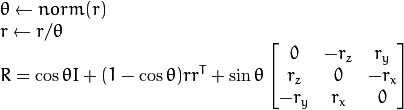# Revision history [back]

### Rodrigues rotation

I do not understand the difference between these two equations:

1. from wikipedia:https://en.wikipedia.org/wiki/Rodrigues%27_rotation_formula

2. from open CV doc:http://docs.opencv.org/2.4/modules/calib3d/doc/camera_calibration_and_3d_reconstruction.html#void%20Rodrigues(InputArray%20src,%20OutputArray%20dst,%20OutputArray%20jacobian)

Where is the cos(θ) gone on the wiki page in the formula 1. ?

Shouln't it be: v_{rot} = cos(θ)v + sin.... ?

Then on the wiki page, there is no more cos(θ) in the definition of R...

Or did I miss something?